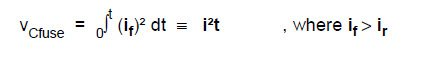# Fuse Model For Over-Current Protection Simulation of DC Distribution Systems.

2.Fuse Model

The fuse model described in this article implements two modelling functions from SPICE derived circuit simulation software; analogue switches and analogue behavioural modelling (ABM). The analogue switch has an output resistance which is controlled by either an input current or voltage. For example, when the input voltage is above 1V the switch is on (low resistance), and when below 0V the switch is off (high resistance), with a transition region occurring when the input is between 0 and 1V. ABM can describe a circuits operation using equations or tables. For instance, an output current can be generated that is equal to an input current squared.
The fuse model, shown in Figure 2, comprises a current controlled switch Wfuse, a voltage controlled voltage source Efuse, a voltage controlled switch Sfuse, and a current controlled current source Gfuse. The model functions by generating a current in Gfuse that is equal to the fuse current squared if², and using this current to charge the capacitor Cfuse. The voltage developed across Cfuse is proportional to i²t and is used to operate Sfuse. The switch Wfuse only allows current to charge Cfuse when the fuse current if is above the fuse rated current ir. The voltage source Efuse operates as a non-linear resistor, modelling the rise in fuse resistance with current due to heating effects. The resistor Rb models the affects of fuse thermal loss by dissipating the voltage across Cfuse. Resistor Ra provides a current path for Gfuse when Wfuse is in an open state, and the RC network Rc/Cc provides a small time delay to ensure one-way operation of the switch Sfuse.

The model accurately represents the following four fuse characteristics: 1) rated current, under which the fuse will not operate; 2) pre-arcing i²t, after which time the fuse will start to operate by limiting current; 3) arcing i²t, after which time the fuse current has reduced to zero; and 4) resistance increase with current, which includes the affects of thermal loss. The expressions describing the fuse model are now presented and have been developed for the commercial software PSpice.

Rated Current : The current controlled switch Wfuse starts to turn on when the fuse current exceeds rated current ir, and is fully on when the fuse current reaches 110% of ir. The model expression for Wfuse is,

.model name iswitch(ION=1.1 ir , IOFF=ir )

Pre-arcing i²t : The voltage developed across Cfuse is,By making the capacitor Cfuse value equal to (i²t)p, in A²s, the voltage developed across Cfuse at the end of the pre-arcing time is normalised to 1V. When the voltage across Cfuse reaches 1V the voltage controlled switch Sfuse starts to turn off, and is fully off when the voltage reaches 1.2V. The model expression for the switch Sfuse is,

.model name vswitch ( VON=1V VOFF=1.2V )

The Rc/Cc network introduces a small time delay to ensure one-way operation of the switch, and the delay can typically be minimised to an insignificant time of less than 0.1msec.

Arcing i²t : The time duration ta for the current to reduce to zero after the onset of arcing can be modelled by the turn-off characteristic of the switch Sfuse. Varying the transition range of the switch Sfuse controlling voltage (VOFF-VON) adjusts the arcing time ta and hence the arcing i²t, (i²t)a.

Resistance Increase and Thermal Loss : During the application of a fault current, the fuse resistance increases with current level as the temperature of the fuse element increases towards the melting point. The resistance increase and resulting voltage drop across the fuse can be substantial. If the fault current is reduced before the i²t level reaches the pre-arcing level (i²t)p , then the fuse element cools and resistance reduces back to the nominal resistance at ambient temperature RF.

The fuse resistance is modelled by a combination of the constant resistance of the switch Sfuse, and the non-linear resistance of the voltage source Efuse. The model expression for the switch Sfuse including resistance is,

.model name vswitch ( RON=RF VON=1V VOFF=1.2V)

The ABM model expression for the voltage source Efuse is,

Efuse <+node> value = { if * vCfuse * (Rf – RF)}

where if is the fuse current, vCfuse is the voltage across the capacitor Cfuse, and Rf is a fuse parameter equal to the fuse resistance at the end of the pre-arcing time.

The thermal loss of the fuse element is modelled by the resistor Rb, which discharges the voltage across capacitor Cfuse. Reducing the value of Rb increases the thermal loss, returning the fuse to its pre-fault state in a faster time.

Parameter Acquisition : The parameters ir, (i²t)p, tp, ip, (i²t)a and ta are readily obtained from manufacturer’s data for the fuse operating in a circuit with a given time constant and prospective current.

The nominal resistance of the fuse RF at ambient temperature can be measured using a 4 terminal milliohm meter or measured in a suitable test circuit, as described in the next section. A measurement of the fuse resistance Rf taken at the end of the pre-arcing time can be made using the test circuit described in the next section.

The inclusion of resistor Rb in the model is optional, as it does not affect fuse operation during a fault leading to fuse operation in a short duration. The affect of Rb is significant only for low levels of fault current or where repetitive fault currents occur with the individual fault level below (i²t)p.

Circuit Breaker Adaptation : The fuse model can be used without modification to represent the operation of circuit breakers, however certain circuit breaker characteristics can only be included by adapting the fuse model. For example, to include the characteristics of minimum operating time, and other special current-time responses, may require the use of ABM using a lookup table.

Posted in OrCAD PSpice and tagged , , , , , .

## One Comment

1. Pingback: Cfuse - Divacheerbows